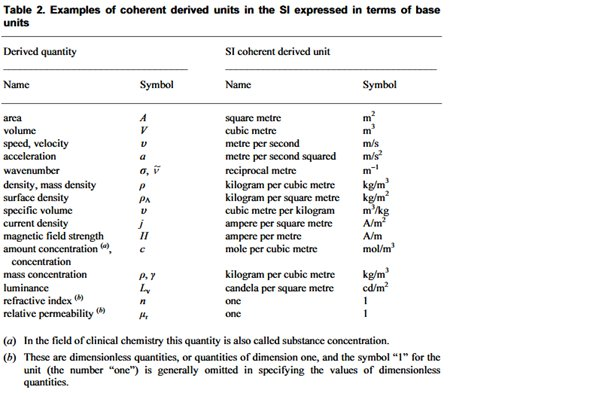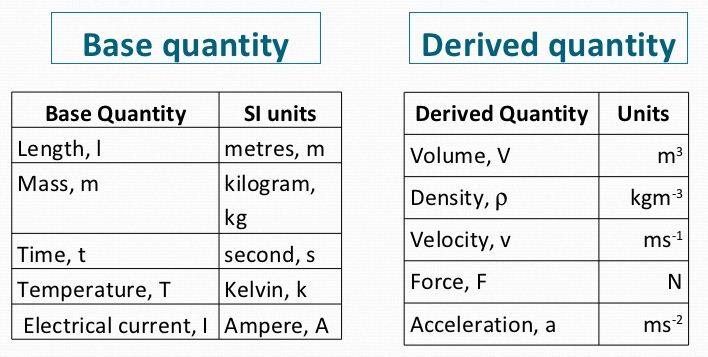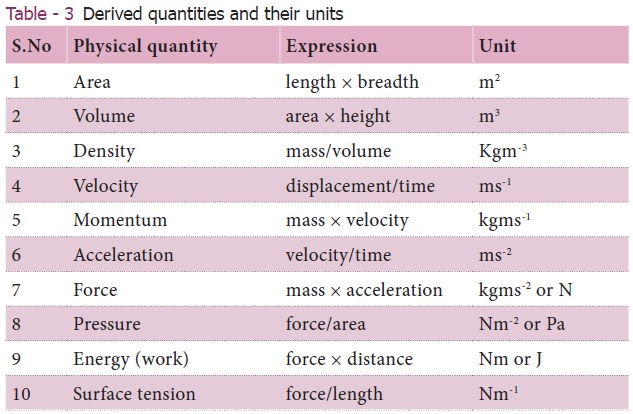Crystal

HS-PS1-3

# Derived Unit Study Guide

Units that are derived from the fundamental units are called derived units. We can obtain these measurement units by multiplying or dividing base units.

Table of content

A base unit is the most elementary unit in the system of measurement. For example, an inch is a unit of length, and a meter is also a unit of length. 'Derived units' are those units that are obtained as a result of a process in which more than one base unit is manipulated with derived units between each other.

## DERIVED UNIT DEFINITION

In SI units, derived units are measure units that have been derived from more than one base unit. The derived units either lack dimension or are the products of the base units. For example, the derived unit of force is Newton (N).

### SYMBOLS AND NAMES OF DERIVED UNIT

Lowercase is used for all derived unit names. In general, the names are combinations of the base units, but there are 22 derived units with special names. Whenever a unit is named after a person, it begins with a capital letter.

### DERIVED UNITS EXAMPLE

The derived units are named after scientists, as some examples, the hertz, the watt, and the coulomb. These units are represented by Hz, W, and C, respectively. In addition to meters per second, cubic meters and joules per kelvin are examples of derived units.

### TOTAL DERIVED UNITS

Radians (radian) and steradians (sr) are among 22 derived units. Additionally, there are over 100 other units whose value is determined by the values of their bases.

### TABLE OF 22 SI DERIVED UNITS### DERIVED UNITS AND DIMENSIONAL ANALYSIS

Dimensional analysis is a method of converting from one unit to another. It is also sometimes called a unit conversion.

In some cases, important to manage estimations that are tiny (as in the size of a molecule) or extremely enormous (as in quantities of iotas). In these cases, it is generally expected important to change over between units of metric estimation. For instance, a mass estimated in grams might be more advantageous to work with on the off chance that it was communicated in mg (10-3g). Changing over between metric units is called unit analysis or dimensional analysis.

There are many other derived units that are mathematical combinations of SI base units but without special names. Some examples are as follows :It is important to note that many derived units lack special symbols.

Dimensional analysis should be used when modifying units or converting them to other units. The process of squaring or cubing units, in this case, area and volume, also squares conversion factors.

As an example, changing cubic meters over to cubic centimeters: (100 cm/1 m)3 = 100 cm3/1 m3 = 1000000 cm3/1 m3

### CONCLUSION

• A derived unit is a unit that outcomes from a numerical combination of SI base units.
• Calculations involving derived units follow similar standards as other unit conversion calculations.

## FAQs

1. What are the 7 derived units?

Seven base SI units are: Length: m (meter) Mass: kg (kilogram) Time: s (second) Electric Current: A (Ampere) Thermodynamic Temperature: K (degrees Kelvin) Amount of Substance: mol (mole) Luminous Intensity: cd (candela)

2. What are derived units? Give an example?

Units that are derived from the fundamental units are called derived units. For example, an inch is a unit of length, and a meter is a unit of length.

3. How many derived units are there?

A total of 22 derived units exist, including the dimensionless radian (radan) and steradian (sr). There are also more than 100 other derived units whose value is expressed in terms of their base units.

4. What are the 10 derived quantities?We hope you enjoyed studying this lesson and learned something cool about Derived Units! Join our Discord community to get any questions you may have answered and to engage with other students just like you! Don't forget to download our App to experience our fun VR classrooms - we promise, it makes studying much more fun! �*